# UniLM: Unified Language Model Pre-training for Natural Language Understanding and Generation

## Basic Idea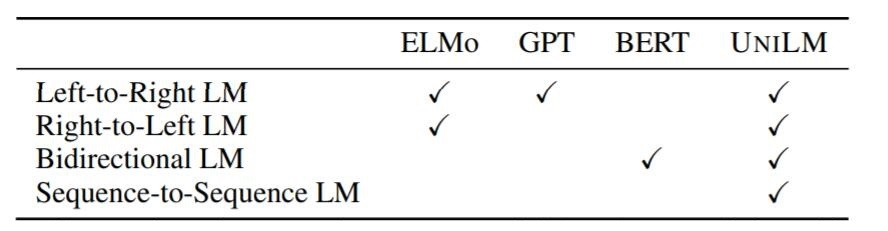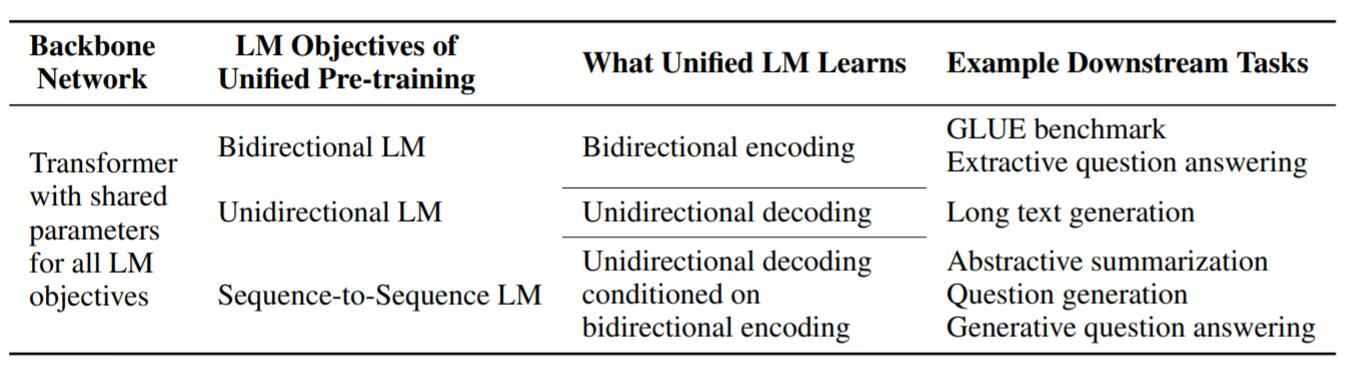## UniLM

### Backbone Network: Multi - Layer Transformer

\begin{aligned} \mathbf{Q} &=\mathbf{H}^{l-1} \mathbf{W}_{l}^{Q}, \quad \mathbf{K}=\mathbf{H}^{l-1} \mathbf{W}_{l}^{K}, \quad \mathbf{V}=\mathbf{H}^{l-1} \mathbf{W}_{l}^{V} \\ \mathbf{M}_{i j} &=\left\{\begin{array}{ll} 0, & \text { allow to attend } \\ -\infty, & \text { prevent from attending } \end{array}\right.\\ \mathbf{A}_{l} &=\operatorname{softmax}\left(\frac{\mathbf{Q} \mathbf{K}^{\top}}{\sqrt{d_{k}}}+\mathbf{M}\right) \mathbf{V}_{l} \end{aligned}

UniLM使用不同的Mask矩阵$\mathbf{M}$ 来决定模型在接下来的运算中能对哪些Token给予注意力. 所以UniLM能直接通过更改$\mathbf{M}$ 在使用同一组参数的条件下改变模型的运行模式, 非常灵活.

### Pre - Training Objectives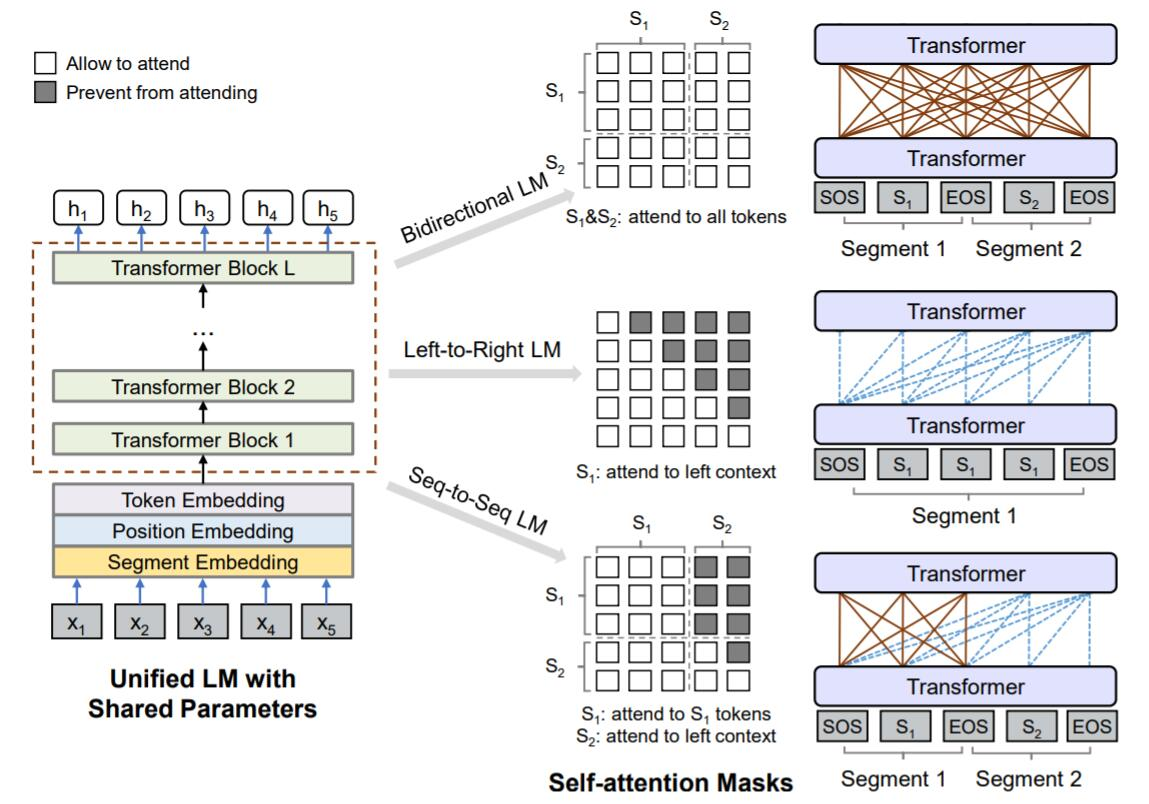UniLM仍然采用BERT类似的完形填空式作为目标, 采用最小化交叉熵的方式来优化模型参数.

• Unidirectional LM - (上图中间): 对于单向语言目标, 不对输入分段, 只输入文本段$\text{S}_1$. 在左到右或右到左的单一方向是时序可见的, 因此只需要将$\mathbf{M}$ 的上三角或下三角设置为$-\infty$, 其余位置置零即可.

• Bidirectional LM- (上图顶端): 对于双向语言目标, 使用了NSP任务, 输入文本段$\text{S}_1, \text{S}_2$, 与BERT训练方式保持一致. Token之间是全部互相可见的, 因此$\mathbf{M}=\mathbf{0}$.

• Sequence-to-Sequence LM - (上图底部): 对于Seq2Seq目标, 输入源序列$\text{S}_1$ 和目标序列$\text{S}_2$, 并在 Encoder对应的$\text{S}_1$ 应该是双向可见的, 故$\mathbf{M}$ 的左侧为$\mathbf{0}$. 在Decoder对应的$\text{S}_2$, 上下文只应该单向可见, 即$\mathbf{M}$ 右侧$\text{S}_2$ 的上三角为$-\infty$, 其余为$\mathbf{0}$.

例如, 在预训练阶段, 对于Seq2Seq任务的输入$\text{S}_1=[t_1, t_2]$, 输出$\text{S}_2=[t_3, t_4, t_5]$, 将以[SOS], $t_1$, $t_2$, [EOS], $t_3$, $t_4$, $t_5$, [EOS] 的形式输入到模型中. $t_2$ 只能看见[SOS], $t_1$, $t_2$, [EOS], 而$t_4$ 可以看到[SOS], $t_1$, $t_2$, [EOS], $t_3$, $t_4$.

在打Mask时, 如果Mask掉的部分为$\text{S}_1$ 的Token, 则有助于模型学到双向Encoder, 若Mask掉的部分为$\text{S}_2$ 的Token, 则有助于让模型学到单向Decoder. 另外, 由于[EOS] 是可以被Mask掉的, 在预测时可以教会模型何时该结束生成.

仔细一想其实挺巧妙的, 因为这样并不需要明确的在模型中划分出Encoder和Decoder的位置. 也就意味着整个模型的所有区域都有可能被当做是Encoder或者Decoder.

### Fine - Tuning on Downstream NLU and NLG Tasks

• 对NLU任务, 采用[SOS] 处的输出作为整个输入的表示, 记为$\mathbf{h}_1^L$, 可以由此计算文本在分类任务上的概率$\operatorname{softmax}\left(\mathbf{h}_{1}^{L} \mathbf{W}^{C}\right)$, $C$ 为类别数, 最大化类别标签的概率即可, 该部分与BERT一致.
• 对NLG任务, 令输入的源序列$\text{S}_1$, 目标序列$\text{S}_2$, 以[SOS], $\text{S}_1$, [EOS], $\text{S}_2$, [EOS] 的形式输入. 训练目标为最大化在给定上下文条件下, 被Mask部分原来内容的概率. 但在精调阶段, 只对$\text{S}_2$ 中的内容打Mask.

## Experiments

### Abstractive Summarization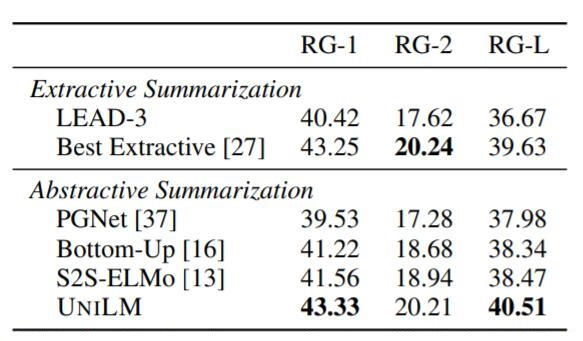Gigaword上生成式摘要结果如下: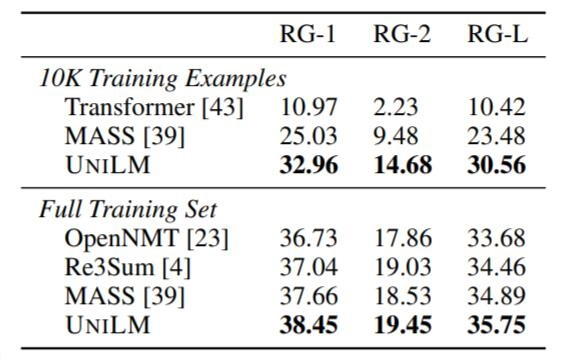UniLM要比Baseline有小幅提升, 并几乎全面领先.

#### Extractive QA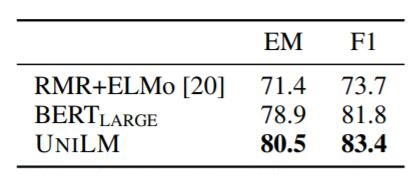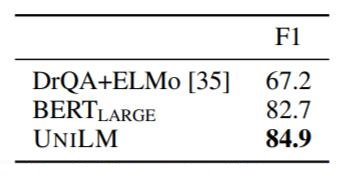#### Generative QA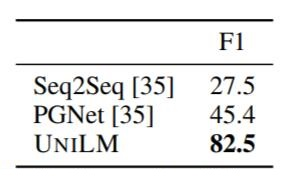### Question Generation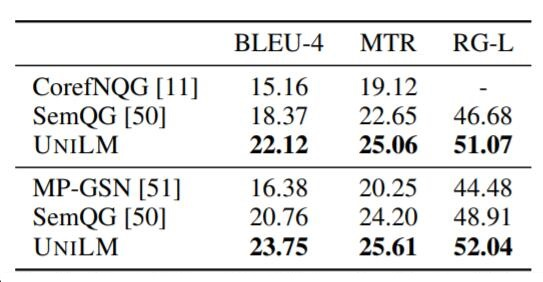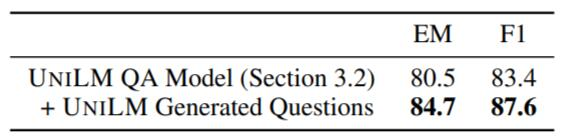### Response Generation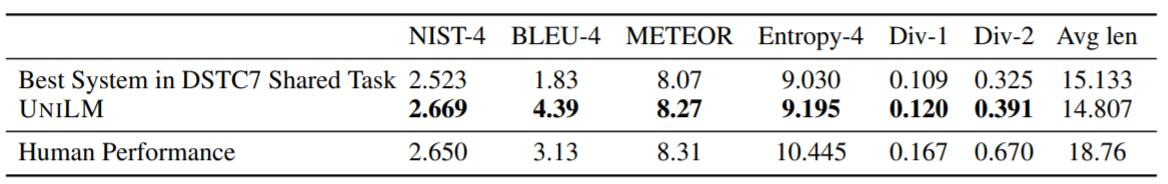### GLUE Benchmark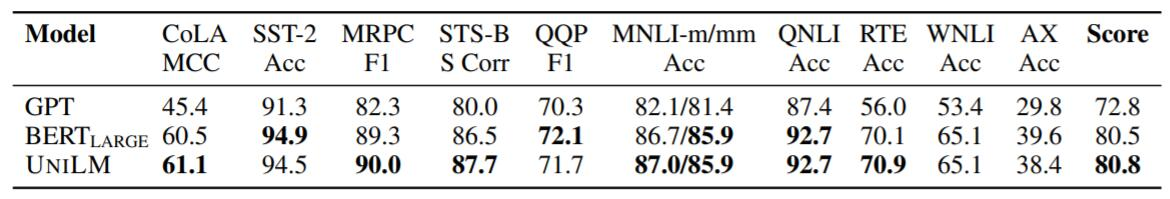UniLM达到了新SOTA.

## Summary

上一篇ALBERT: A Lite BERT for Self-supervised Learning of Language Representations

2021-06-29MASS: Masked Sequence to Sequence Pre - training for Language Generation

2021-06-08
目录